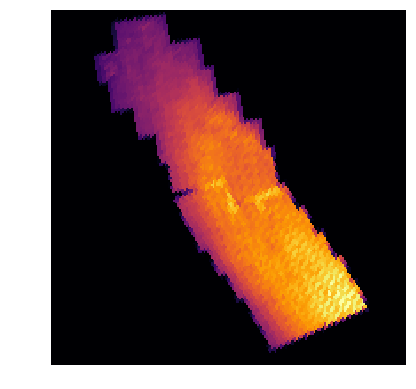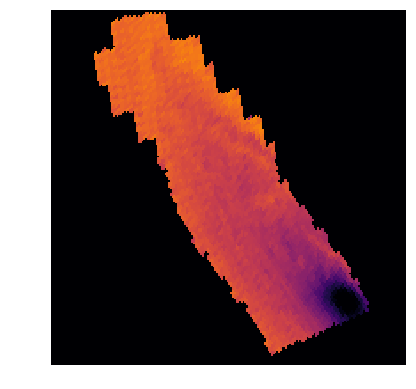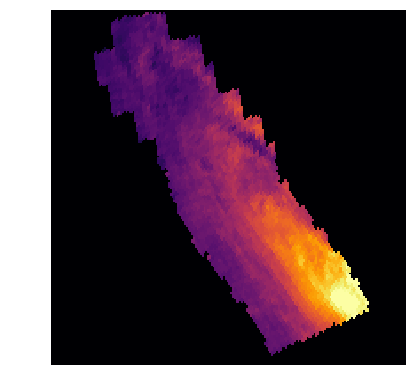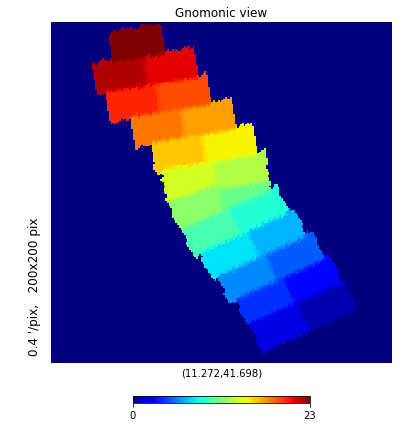In :
__author__ = 'Knut Olsen <kolsen@noao.edu>' # single string; emails in <>
__version__ = '20180103' # yyyymmdd; version datestamp of this notebook
__datasets__ = ['phat_v2']  # enter used datasets by hand


# Fun with PHAT!¶

The Panchromatic Hubble Andromeda Treasury (PHAT; PI Dalcanton) is a Hubble Space Telescope Multi-cycle program to map roughly a third of M31's star forming disk, using 6 filters covering from the ultraviolet through the near infrared.

The Data Lab hosts two main tables from PHAT: the version 2 object table (phat_v2.phot_mod) and the version 2 single epoch measurement table (phat_v2.phot_meas). The object table contains average photometry in all filters, with one row per object. The measurement table contains all of the photometric measurements for all objects, with one row per measurement. The measurement table contains ~7.5 billion rows.

In this notebook, we'll use these tables to do some exploration of the PHAT survey.

If you use this notebook for your published science, please acknowledge the following:

# Imports and setup¶

In :
import numpy as np
import pylab as plt
import matplotlib
import healpy as hp

%matplotlib inline

# Datalab and related imports
from dl import authClient as ac, queryClient as qc, helpers


# Authentication¶

In :
# Python 2/3 compatibility
try:
input = raw_input
except NameError:
pass

# Either get token for anonymous user


# Basic info¶

First, let's look at the tables available in the PHAT database, and then get some basic information from the Data Lab statistics database (tbl_stat) about the main PHAT object table, phat_v2.phot_mod.

In :
try:
print(qc.schema('phat_v2',format='json',profile='default'))
except Exception as e:
print(e.message)

Schema: phat_v2

Table Name   Description
----------   -----------
phot_meas
phot_mod
phot_v2
star_v2


In :
%%time
query = "SELECT * FROM tbl_stat WHERE schema='phat_v2' and tbl_name='phot_mod'" # Retrieve useful stats, quickly
try:
info = qc.query(token,sql=query) # by default the result is a CSV formatted string
except Exception as e:
print(e.message)

CPU times: user 20 ms, sys: 4 ms, total: 24 ms
Wall time: 69.5 ms

In :
print(info)

schema,tbl_name,nrows,ncols,nindex,table_size,indexes_size,total_size
phat_v2,phot_mod,118774088,88,44,43 GB,102 GB,145 GB



# Examine the columns of the PHAT object table¶

First, we'll take a look at some rows from phat_v2.phot_mod, and get all columns.

In :
query = """SELECT *
FROM phat_v2.phot_mod
LIMIT 100
"""

In :
%%time
try:
result = qc.query(token,sql=query) # by default the result is a CSV formatted string
except Exception as e:
print(e.message)

CPU times: user 23 ms, sys: 2 ms, total: 25 ms
Wall time: 81.2 ms


### Convert the output to a Pandas Dataframe¶

Pandas dataframes are a convenient way to store and work with the data. The Data Lab 'helpers' module (docs) has a conversion method, with many possible output formats.

In :
df1 = helpers.convert(result,'pandas')
print("Number of rows:", len(df1))
print(len(df1.columns))

Returning Pandas dataframe
Number of rows: 100
Index(['ra', 'dec', 'htm9', 'pix256', 'pix4096', 'random_id', 'x', 'y', 'ir_x',
'ir_y', 'f110w_rate', 'f110w_raterr', 'f110w_vega', 'f110w_err',
'f110w_chi', 'f110w_snr', 'f110w_sharp', 'f110w_round', 'f110w_crowd',
'f160w_rate', 'f160w_raterr', 'f160w_vega', 'f160w_err', 'f160w_chi',
'f160w_snr', 'f160w_sharp', 'f160w_round', 'f160w_crowd', 'f275w_rate',
'f275w_raterr', 'f275w_vega', 'f275w_err', 'f275w_chi', 'f275w_snr',
'f275w_sharp', 'f275w_round', 'f275w_crowd', 'f336w_rate',
'f336w_raterr', 'f336w_vega', 'f336w_err', 'f336w_chi', 'f336w_snr',
'f336w_sharp', 'f336w_round', 'f336w_crowd', 'f475w_rate',
'f475w_raterr', 'f475w_vega', 'f475w_err', 'f475w_chi', 'f475w_snr',
'f475w_sharp', 'f475w_round', 'f475w_crowd', 'f814w_rate',
'f814w_raterr', 'f814w_vega', 'f814w_err', 'f814w_chi', 'f814w_snr',
'f814w_sharp', 'f814w_round', 'f814w_crowd', 'f814w_f475w',
'f475w_f336w', 'f336w_f275w', 'f275w_f160w', 'f160w_f110w',
'f110w_flag', 'f160w_flag', 'f275w_flag', 'f336w_flag', 'f475w_flag',
'f814w_flag', 'f275w_gst', 'f336w_gst', 'f475w_gst', 'f814w_gst',
'f110w_gst', 'f160w_gst', 'inside_ir', 'inside_brick', 'inside_chipgap',
'inside_other_chipgap', 'field', 'brick', 'objid'],
dtype='object')
88


# Make an object density map¶

One of the columns in the PHAT object table, pix4096, is the Healpix index (NSIDE=4096, nested scheme) for the objects's RA and Dec. Healpix is a handy tesselation of the sky into tiles of equal area. The Python module healpy has all of the Healpix related functions.

To make maps of aggregate quantities in PHAT, we're going to use the database to return results in a query grouped by Healpix index value. We can then put the results into arrays, and use healpy's functionality to display the maps.

In this first query, the GROUP BY clause tells the database to aggregate the results by the values in the pix4096 column, and return the average RA and Dec of objects in those groups, as well as the pix4096 value itself and the count of the number of objects in the group.

In :
query = """SELECT avg(ra) as ra0,avg(dec) as dec0,pix4096,count(pix4096) as nb
FROM phat_v2.phot_mod
GROUP BY pix4096
"""

In :
%%time
# This query should take about a minute
try:
result = qc.query(token,sql=query) # by default the result is a CSV formatted string
except Exception as e:
print(e.message)

CPU times: user 29 ms, sys: 1 ms, total: 30 ms
Wall time: 41.6 s


### Convert the output to a Pandas Dataframe¶

We'll once again use helpers to convert the result to a Pandas dataframe. Our dataframe has one row per Healpix. We'll check that the sum of the number of objects in all Healpixels equals the number of rows in the table.

In :
df_density = helpers.convert(result,'pandas')
print("Number of rows:", len(df_density))
print(np.sum(df_density['nb'])) # print total counts

Returning Pandas dataframe
Number of rows: 2574
117335533


### Making the Healpix map¶

A Healpix map is simply a one-dimensional array with number of elements set by the NSIDE parameter, which is the number of times the base Healpixels are split. We can visualize it as a map using the healpy library.

In :
hmap = np.zeros(hp.nside2npix(4096))

In :
print(df_density.head())

         ra0       dec0   pix4096     nb
0  11.239025  41.610492  10747797  68020
1  11.294279  41.722430  10747868  63630
2  11.693241  41.890020  10922465  12500
3  11.457172  41.810363  10922601  51534
4  11.843336  42.047941  10923119  22544


### Populating the Healpix map¶

Now we set the elements of our Healpix map to the number of objects returned by the query, calculate the center of the RA and Dec distribution of the objects, and use healpy's gnomview to visualize the output. Notice anything funny? The PHAT object table has duplicate objects between some of the bricks (bricks 11, 12, 13, and 14).

In :
hmap[df_density['pix4096']]=df_density['nb']
(rarot,decrot)=(np.median(df_density['ra0']),np.median(df_density['dec0']))

In :
hp.gnomview(hmap,title='',notext=True,cbar=False,reso=0.4,nest=True,rot=(rarot,decrot,0),cmap='inferno',min=1e3,max=8e4)# Make depth and color maps¶

Now we'll get a little fancier with our maps. We'll have the database return average WFC3 u-band and ACS magnitudes and colors and the PHAT brick numbers, and make some cuts on the magnitudes and flags of the objects. We again GROUP BY the pix4096 column.

In :
query = """SELECT avg(ra) as ra0,avg(dec) as dec0,pix4096,count(pix4096) as nb,
avg(f475w_vega) as gmag,avg(f814w_vega) as imag,avg(brick) as brick,
avg(f475w_vega-f814w_vega) as g_i
FROM phat_v2.phot_mod
WHERE f475w_gst=1 AND f814w_gst=1 AND f475w_vega<50 AND f814w_vega<50
GROUP BY pix4096
"""
print(query)

SELECT avg(ra) as ra0,avg(dec) as dec0,pix4096,count(pix4096) as nb,
avg(f475w_vega) as gmag,avg(f814w_vega) as imag,avg(brick) as brick,
avg(f475w_vega-f814w_vega) as g_i
FROM phat_v2.phot_mod
WHERE f475w_gst=1 AND f814w_gst=1 AND f475w_vega<50 AND f814w_vega<50
GROUP BY pix4096


In :
%%time
# Query will take 1 - 2 minutes
try:
result = qc.query(token,sql=query) # by default the result is a CSV formatted string
except Exception as e:
print(e.message)

CPU times: user 171 ms, sys: 10 ms, total: 181 ms
Wall time: 1min 29s

In :
df_all = helpers.convert(result,'pandas')
print("Number of rows:", len(df_all))
print(np.sum(df_all['nb'])) # print total counts

Returning Pandas dataframe
Number of rows: 2574
81469723

In :
print(df_all.head())

         ra0       dec0   pix4096     nb       gmag       imag      brick  \
0  11.238961  41.610199  10747797  42598  26.615453  24.934838  10.085027
1  11.294243  41.722467  10747868  43786  26.615977  24.997110  12.447312
2  11.693173  41.890007  10922465   7543  27.030190  25.735297  18.000000
3  11.456964  41.810426  10922601  34910  26.747490  25.257324  15.918763
4  11.842830  42.048000  10923119  11078  26.964323  25.645896  20.000000

g_i
0  1.680615
1  1.618867
2  1.294892
3  1.490165
4  1.318427


### Healpix map of average F475W magnitude¶

The map of the average F475W magnitude gives a good idea of how the PHAT catalog depth varies with position in M31. The depth is much shallower in the Bulge, which is very crowded, than in the outer disk.

In :
gmap = np.zeros(hp.nside2npix(4096))
gmap[df_all['pix4096']] = df_all['gmag']
hp.gnomview(gmap,title='',notext=True,cbar=False,reso=0.4,nest=True,rot=(rarot,decrot,0),cmap='inferno',min=25,max=28)### Healpix map of average F475W-F814W color¶

The map of average color reveals both population differences and the dust lanes in the galaxy, as well as the 10 kpc ring.

In :
gimap = np.zeros(hp.nside2npix(4096))
gimap[df_all['pix4096']] = df_all['g_i']
hp.gnomview(gimap,title='',notext=True,cbar=False,reso=0.4,nest=True,rot=(rarot,decrot,0),cmap='inferno',min=1,max=2.5)### Healpix map of the brick number¶

We can also use our Healpix table to make a map of the PHAT bricks.

In :
brickmap = np.zeros(hp.nside2npix(4096))
brickmap[df_all['pix4096']] = df_all['brick']
hp.gnomview(brickmap,reso=0.4,nest=True,rot=(rarot,decrot,0),cmap='jet',min=0,max=23)# Do a spatial query and make color-color diagrams and CMDs¶

Now let's do a cone search for objects within a radius of a particular position. The PHAT tables are spatially indexed to make such queries fast. We'll search within a 1 arcmin radius of the RA and Dec position that we defined earlier.

In :
query = """SELECT *
""".format(rarot,decrot,1./60)
print(query)

SELECT *


In :
%%time
try:
result = qc.query(token,sql=query) # by default the result is a CSV formatted string
except Exception as e:
print(e.message)

CPU times: user 904 ms, sys: 338 ms, total: 1.24 s
Wall time: 9.64 s


### Cut out bad missing values¶

Some of the objects returned will have missing magnitude measurements, indicated by 99's. Let's cut those out, and also select only the "good" stars in the ACS bands.

In :
df = helpers.convert(result,'pandas')
print("Number of rows:", len(df))
df_cmd = df[(df['f336w_vega']<50) & (df['f475w_vega']<50) & (df['f814w_vega']<50) & \
(df['f475w_gst']==1) & (df['f814w_gst']==1)]
print("Number of rows:", len(df_cmd))

Returning Pandas dataframe
Number of rows: 246439
Number of rows: 97786


### Make color-color and CMD plots¶

We'll show the F475W-F814W,F336W-F475W color-color diagram, and a color-magnitude diagram. What do you notice?

In :
# make a figure
fig = plt.figure(figsize=(20,10))

# color-magnitude diagram (Hess diagram)
im = ax1.hexbin(df_cmd['f475w_vega']-df_cmd['f814w_vega'],df_cmd['f336w_vega']-df_cmd['f475w_vega'], \
gridsize=400,cmap=matplotlib.cm.viridis,norm=matplotlib.colors.LogNorm())
ax1.set_ylabel('F336W - F475W',fontsize=15)
ax1.set_xlabel('F475W - F814W',fontsize=15)
ax1.set_title('Color-color diagram',fontsize=20)
ax1.set_ylim(-2,6)
ax1.set_xlim(-1,5)

# color-magnitude diagram (Hess diagram)
im2 = ax2.hexbin(df_cmd['f475w_vega']-df_cmd['f814w_vega'],df_cmd['f475w_vega'], \
gridsize=200,cmap=matplotlib.cm.viridis,norm=matplotlib.colors.LogNorm())
ax2.set_xlabel('F475W - F814W',fontsize=15)
ax2.set_ylabel('F475W',fontsize=15)
ax2.set_title('Color-magnitude (Hess) diagram',fontsize=20)
ax2.set_xlim(-1,5)
ax2.set_ylim(28.4,22)

Out:
(28.4, 22)